﻿ NRectangleF Structure Members
Nevron .NET Vision
Nevron.GraphicsCore Namespace / NRectangleF Structure
Fields Properties Methods

In This Topic
NRectangleF Structure Members
In This Topic

The following tables list the members exposed by NRectangleF.

Public Constructors
NameDescription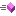Overloaded.
Top
Public Fields
NameDescription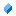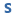The empty rectangleRectangle heightRectangle widthX coordinate of the left top cornerY coordinate of the left top corner
Top
Public Properties
NameDescription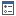Obtains the rectangle area (width * Height)Gets the bottom side of the rectangleGets the minimal enclosing rectangleGets the minimal enclosing 2D rangeGets/Sets the rectangle center.Determines whether this is a flat primitiveGets the rectangle left bottom cornerGets/sets the rectangle location (left-top corner)Gets an array with the rectangle sides middle points, enumerated in a top, right, bottom and left order.Obtains the rectangle perimeter (2 * width) + (2 * Height)Gets the right side of the rectangleGets the rectangle right bottom cornerGets the rectangle right top cornerGets/sets the size of this rectangleGets an array with the rectangle vertices, enumerated in a top, right, bottom and left order.Gets an array with the rectangle vertices and middles enumerated clockwise starting from the left-top vertex
Top
Public Methods
NameDescriptionAppends the primitive to the specified graphics pathDetermines whether this rectangle intersects with the specified circleOverloaded. Determines whether the rectangle contains the specified pointOverloaded. Determines whether the outline of the rectangle contains the specified pointReturns the fitted rectangle with the specified orientation (in degrees)Overloaded. Gets a rectangle from center and dimensionsGets a rectangle from the coordinates of it's sidesOverloaded. Gets a normalized rectangle from two pointsObtains the point on the rectangle rim, which corresponds to the specified compass directionObtains the point on the rectangle rim, which corresponds to the specified compass directionIncludes the point in this rectangleOverloaded. Determines whether the rectangle contains the point with the specified coordinatesDetermines whether this rectangle intersects with the specified rectangleOverloaded. Inflates the rectangle with the specified amountsOverloaded. Returns a third rectangle that represents the intersection of two rectangles. If there is no intersection, the Empty rectangle is returned.Computes the intersection area of this rect with the specified rectOverloaded. Determines whether this rectangle intersects with the specified rectangleIntersects this rectangle with the specified line segmentIntersects this rectangle with a line connecting the rectangle center and the specified pointIntersects this rectangle with the ray from the bounds central pointNormalizes this rectangleDetermines whether this rectangle outline intersects with the passed rectComputes a new rect for an expected resize of a rectangle in the specified compass directionRound this rectangleScales the rectangle with the specified factorsConverts this rectangle to NRange2DFConverts this rectangle to RectangleConverts this rectangle to RectangleConverts this rectangle to RectangleFReturns the string representation of this objectOverloaded. Translates the primitive with the specified amountsOverloaded. Creates the smallest possible third rectangle that can contain the two rectangles that form a union.
Top
Public Operators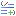Equality Determines whether a is equal to bInequality Determines whether a is not equal to b
Top